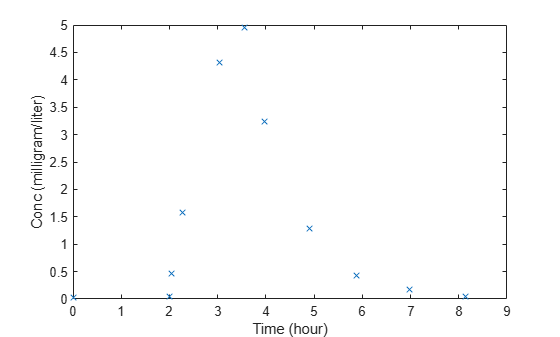LagParameterName

Parameter specifying time lag for dose

Description

LagParameterName is a property of a RepeatDose or ScheduleDose object.

Specify the name of a parameter object that is scoped to a model. The parameter defines the length of time it takes for the dose to reach its target after being introduced.

You can parameterize the property by setting it to the name of a model-scoped parameter that is not being modified by a repeated assignment rule, an algebraic rule, or a rate rule. However, the parameter can be modified by an event.

Characteristics

 Applies to Objects: RepeatDose, ScheduleDose. Data type Character vector. Data values Name of a model-scoped parameter object. The default value is an empty character vector ''. Access Read/write.

Examples

expand all

This example shows how to estimate the time lag before a bolus dose was administered and the duration of the dose using a one-compartment model.

Plot the data.

plot(data.Time,data.Conc,'x')
xlabel('Time (hour)')
ylabel('Conc (milligram/liter)')Convert to groupedData.

gData = groupedData(data);
gData.Properties.VariableUnits = {'hour','milligram/liter'};

Create a one-compartment model.

pkmd                    = PKModelDesign;
pkc1.DosingType         = 'Bolus';
pkc1.EliminationType    = 'linear-clearance';
pkc1.HasResponseVariable = true;
model                   = construct(pkmd);
configset               = getconfigset(model);
configset.CompileOptions.UnitConversion = true;

Add two parameters that represent the time lag and duration of a dose. The lag parameter specifies the time lag before the dose is administered. The duration parameter specifies the length of time it takes to administer a dose.

lagP.ValueUnits = 'hour';
durP.ValueUnits = 'hour';

Create a dose object. Set the LagParameterName and DurationParameterName properties of the dose to the names of the lag and duration parameters, respectively. Set the dose amount to 10 milligram which was the amount used to generate the data.

dose                = sbiodose('dose');
dose.TargetName     = 'Drug_Central';
dose.StartTime      = 0;
dose.Amount         = 10;
dose.AmountUnits    = 'milligram';
dose.TimeUnits      = 'hour';
dose.LagParameterName = 'lagP';
dose.DurationParameterName = 'durP';

Map the model species to the corresponding data.

responseMap = {'Drug_Central = Conc'};

Specify the lag and duration parameters as parameters to estimate. Log-transform the parameters. Initialize them to 2 and set the upper bound and lower bound.

paramsToEstimate    = {'log(lagP)','log(durP)'};
estimatedParams     = estimatedInfo(paramsToEstimate,'InitialValue',2,'Bounds',[1 5]);

Perform parameter estimation.

fitResults = sbiofit(model,gData,responseMap,estimatedParams,dose,'fminsearch')
fitResults =
OptimResults with properties:

ExitFlag: 1
Output: [1x1 struct]
GroupName: One group
Beta: [2x4 table]
ParameterEstimates: [2x4 table]
J: [11x2 double]
COVB: [2x2 double]
CovarianceMatrix: [2x2 double]
R: [11x1 double]
MSE: 0.0024
SSE: 0.0213
Weights: []
LogLikelihood: 18.7511
AIC: -33.5023
BIC: -32.7065
DFE: 9
DependentFiles: {1x2 cell}
EstimatedParameterNames: {'lagP'  'durP'}
ErrorModelInfo: [1x3 table]
EstimationFunction: 'fminsearch'

Display the result.

fitResults.ParameterEstimates
ans=2×4 table
Name      Estimate    StandardError    Bounds
________    ________    _____________    ______

{'lagP'}     1.986        0.0051568      1    5
{'durP'}     1.527         0.012956      1    5

plot(fitResults)